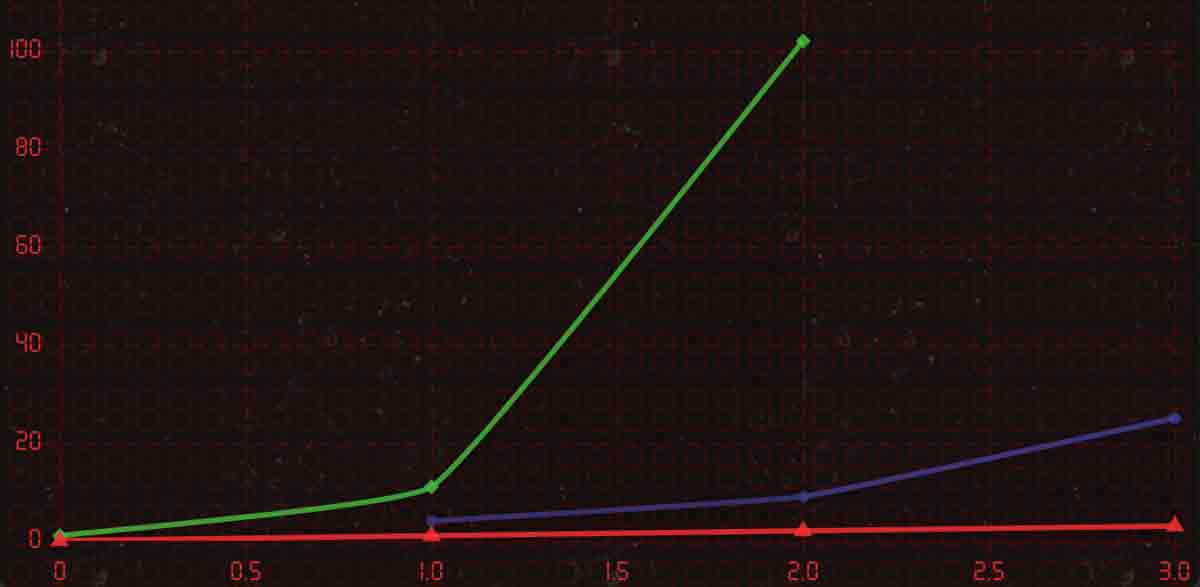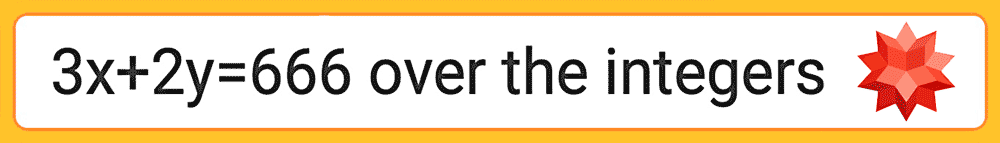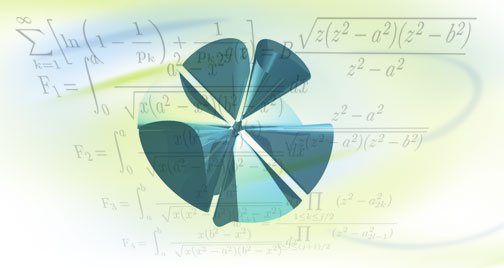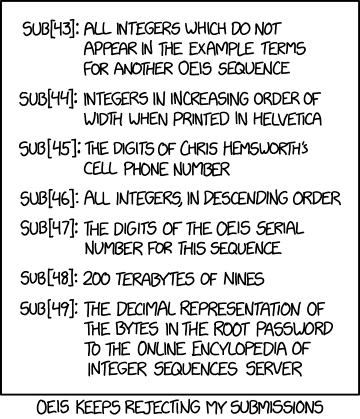useR Guide tHe deVil's cAlculAtoR

hoW to plAy In the The Devil's Calculator, all normal arithmetic operators (addition, multiplication, etc...) are replaced by a strange diamond symbol, "◇". This represents an evil mathematical operation, a function that does something unknown to its input, and it becomes more difficult and obscure each level.

5◇=6
◇ is adding one to the input
expressed ◇=f(x)=x+1

You objective is, through interpolation, to uncover the "◇" function and then work backwards to use it in calculating 666. Once the number 666 has been calculated using the ◇ key, the level is won. Think of it like the typical scenario of solving for x in an algebraic expression, but the operators themselves are unknown, rather than a constant. To add to the challenge, numbers will occasionally be on fire and you will be unable to use them to get data on operands or to calculate 666.

Creator Christopher Jarvis introduces the game at the MoMath MOVES conference

opeRAtoRs As you progress in the game, new mathematical operators are unlocked. There are three, in total. "◇" is a unary operator, (much like a square root), that takes in one input and returns a number. "ψ" is a binary operator, (like addition or division) that takes in two inputs and returns a number. Finally, "ф" is a unary operator that takes an a positive integer as its input, and returns the index of a mystery integer sequence. If "ф" represents prime numbers, 3ф=5 since 5 is the third prime. To conquer the game, you will need to use all three operators together to calculate 666. "ф" will always return an integer and will only accept an integer as an input

◇ф(3ψ2)=666
◇=x*111 | ψ=x^y | ф=digit of pi
3^2 is 9, 9th digit of pi is 6, 6*111=666

negAtion An early mechanic that deserves a mention is negation. If you have the following expression, pressing the ± button will toggle between four states, where the result of the function is negated, the input is negated, and both the input and the resulting function are negated.

◇2 -◇2 ◇-2 -◇-2
All four are very different

Recursion An important mechanic that becomes apparent rather early, (in level 5) is recursion. Functions can and will need to be used many times in succession. Suppose ◇=a^2. ◇3 would therefore be 9 and ◇◇3 would be 81, or ◇9. What would ◇◇◇3 be? Parentheses can make it clearer: (◇(◇(◇3))) means the expression would be 6561 or ◇81.

◇◇◇◇2
if ◇=2a-1:
2(2(2(2*2-1)-1)-1)-1 = 17

pARentheses It is important to remember that all operators need to be used in the service of calculating 666, else the level will not be won. There are some levels where it is trivially easy to calculate 666 with one function, but the difficulty comes with incorporating another. Another twist is parentheses and order of operations: ◇(3 ψ 5) is very different from ◇3 ψ 5. Let's say ◇=a+2 ψ=a*b. In the latter example, the ◇ function will first act on the 3, and its result (5) will then be the first input of the be the ψ function, resulting in 5*5=25. In the first example, the ψ would go first, (3*5=15) and then the ◇ function would act on its result. 15+2=17. You'll notice there is no button for parentheses - that is because they are added automatically depending on the order in which the operators are added in the equation. In keeping with our earlier example, if you key in 3 ψ 5 and then press the ◇ button, it will automatically prepend the ◇ function and parentheses to the binary expression.

◇((5ψ◇7)ψ1)
Order of operations:
a=◇7, b=5ψa, c=bψ1, answer=◇c

difficulty This game is meant to live up to its name. Particularly later levels can be fiendishly difficult. If you find yourself struggling, you can always skip a level and return later. Consult the "help" button often, it has different advice for every level in the game. Look to the steam community or Twitch | YouTube let's plays for guidance, and feel free to reach out on twitter: @devilscalc.

Mathematician James Tanton introduces the concepts of The Devil's Calculator

Graphing Graphing can be a valuable asset for inferring patterns in data. (There is now support for pan, scan and zoom). Each of the three operators are demarcated by different colors (red, green, blue) and their point shapes. Graphing can sometimes be deceptive as many challenges are non-algebraic. Hitting the "p" key will copy your formula history to the clipboard and is formatted as CSV so you can bring it into your favorite spreadsheet application and customize your graphs / line fit.Graphing can offer important insights

wolfrAM AlphA Wolfram is deeply integrated within the game. To query wolfram alpha, go to the "notes" menu, and tap the 'wolfram' button to input a search. As the game is more logic based, you are not expected to do algebra (particularly multivariate algebra) and using Wolfram Alpha with the flag "over the integers" can save enormous amounts of time. If you determine that ψ=(a+5)/b, then you can type "(a+5)/b=666 over the integers" and wolfram will list integer pairs. This is also helpful for recursive functions: if ◇=3a+5, you can test if there is an integer answer for ◇◇X=666 by typing in "3*(3a+5)+5=666 over the integers." You can also enter the field blank, to upload your notes for Natural Language Processing.The over the integers flag is a lifesaver
if you suspect your answer is an integer

Holding down the screen in the "help" or "hint" menu will open up unique links to Wolfram MathWorld to point you in the right direction on the puzzle. Holding down the graph screen will open a scatterplot of your current data on wolfram alpha. Holding down the screen in the "notes" menu will open wolfram notebooks for you to better organize your strategies with the level. Created levels will soon support links to Wolfram cloud API functions. A helpful trick with the "over the integers" flag is the following: Floor(a*100)/100=666 over the integers will provide answers up to two decimal points.Wolfram MathWorld is a mathematical encyclopedia that has entries for virtually every type of puzzle in the game

otheR tools Players can access a scientific calculator provided by Desmos either by going into the "notes" menu or holding the screen down. This is important since you are not expected to do computations manually. A link to OEIS becomes available once its operator is unlocked, and consulting its database is recommended for deciphering the logic behind a level.xkcd is the best

MeMoRy keys Memory keys are an important tool and their utility may not be immediately obvious. In addition to being able to keep specific calculations handy if needed, their real usefulness is in state management. Once you unlock multiple operators, you'll need to use all three together to calculate 666. You can compartmentalize this task using memory keys, since saving a calculation to a memory key saves the operators used in that calculation (why the buttons light up). This way you can avoid having to build a long equation from scratch and instead build upon work you've saved over the course of the level.

◇5=74 → M1 : M1ψ9=666
You can save the result 74 along with the ◇ state for easy recall

dlc | online Mode In the "graph" menu, there is the "levels" button. This allows you to replay levels from the regular game and to check if there is new DLC. The next DLC will automatically load when pressed. You may skip the level and return to it anytime using the replay menu. if there are no new DLC levels, you have the option of playing community challenges even if you have not yet beaten the game, though this is discouraged, as the concepts introduced later in the game may be necessary for success.DLC levels can prove even more
challenging than the regular game.

level cReAte Anyone can suggest levels for DLC inclusion here, but from within the game, you can build and upload levels without moderation. Click "graph" -> "levels" -> "create" and you will be prompted for a username. Once inputted, you can design levels using math.js between ternary if conditions. "a" is always the first input of a function, and "b" if there is a second.

a%2 ? a/2 : a+3
will divide by 2 when the input is
odd and add 3 when even

Everything between ternary operators will be fed into math.eval, which supports simple expressions and advanced functionality. For questions, contact us on twitter: @devilscalc, or submit a level via our online form and we can code it for you.

a==b ? a*b : a/b
will multiply both inputs together
when they are the same, and divide them when different

Integer sequence functions can be generated with a manual series or array of numbers i.e. '[0,1,1,2,3,5,8,13,21,34][a]' would be the start of Fibonacci, or you can compute them dynamically:"

string(pi)[a]
will return the (a)th digit of pi

AdVAnced tRicks Hold '+/-' or type '_' on desktop to mute sound effects. On mobile, enter '.0900' followed by equals to supress haptics. Enter '.000' followed by equals to clear online level data. Type '.00000' followed by equals to reset your game data (purchase history will remain). You can jump quickly from level to level by entering a period, followed by the level number, another period, and then hitting equals. i.e. '.XX.' will take you to level XX. To skip the entire game and go directly to the postgame, you can enter .4973. followed by equals. For desktop, a list of keyboard shortcuts is below:

Δ: a,j,[
ψ: s,k,]
φ: d,l,\\
Equals: Enter, =
Clear: backsp,esc, c
AC: Delete, shift+C
Calc: #
help: right arrow
notes:left arrow
Wolfram: w , OEIS: o
MR1: shift+1
MR2: shift+2
MR3: shift+3
MS1: alt+1
MS2: alt+2
MS3: alt+3
SFX/Mute: _
graph: up/dn arrow
Server Sync: *
CopyClip: pCinq-Mars Media a 501(c)3 non-profit
Cinq-Mars Media is a 501(c)(3) non-profit organization developing technology to support scientific research related to human safety and education, and to make such technology and research available on a charitable basis. *Cinq-Mars Media is not affiliated with Numberphile and does not endorse their content. All content copyright the respective owners. The Devil’s Calculator © Cinq-Mars Media 2018